# ISEE Upper Level Math : How to find the perimeter of other polygons

## Example Questions

### Example Question #1 : Other Polygons

If the perimeter of a septagon is equal to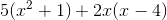, what is the length of one side? (All sides of the septagon are equal.)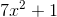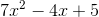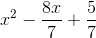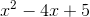Explanation:

If the perimeter of a septagon (in which all sides are equal) is, then the length of one side will be one seventh of this expression.

To find one seventh, the value must first be simplified and then divided by 7.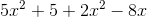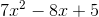When this is divided by 7, the result is:This value is thus the length of one side of the septagon.